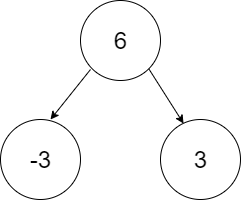New update is available. Click here to update.

# Sum Paths

Last Updated: 26 Feb, 2021
Difficulty: Moderate

## PROBLEM STATEMENT

#### Note :

``````A path may or may not start at the root of the tree. A path may or may not end on a leaf node. You are allowed to travel only downwards. This means after visiting any node, you are allowed to visit only its children.
``````

#### Input Format :

``````The first line contains an integer 'T', which denotes the number of test cases or queries to be run. Then, the 'T' test cases follow.

The first line of each test case contains a single integer, 'Target', as described in the problem statement.

The second line of each test case contains elements of the binary tree in the level order form. The line consists of values of nodes separated by a single space. In case a node is null, we take -1 in its place.
``````

#### For example, the input for the tree depicted in the below image would be :``````6
-3 3
-1 -1 -1 -1
``````

#### Explanation :

``````Level 1 :
The root node of the tree is 6

Level 2 :
Left child of 6 = -3
Right child of 6 = 3

Level 3 :
Left child of -3 = null (-1)
Right child of -3 = null (-1)
Left child of 3 = null (-1)
Right child of 3 = null (-1)

The first not-null node (of the previous level) is treated as the parent of the first two nodes of the current level. The second not-null node (of the previous level) is treated as the parent node for the next two nodes of the current level and so on.
The input ends when all nodes at the last level are null (-1).
``````

#### Note :

``````The above format was just to provide clarity on how the input is formed for a given tree.

The sequence will be put together in a single line separated by a single space. Hence, for the above-depicted tree, the input will be given as:

6 -3 3  -1 -1 -1 -1
``````

#### Output Format :

``````For each test case, print an integer, denoting the number of different paths such that the sum of values of nodes in each path equals K.

Output for each test case will be printed in a separate line.
``````

#### Note :

``````You do not need to print anything. It has already been taken care of. Just implement the given function.
``````

#### Constraints :

``````1 <= T <= 100
1 <= N <= 5000
1 <= Target <= 10^9
-10^9 <= node data <= 10^9, , (where node data != -1).

Time Limit: 1sec
``````## Approach 1

Approach:

For any node, the number of paths will be equal to the sum of the number of such paths starting from this node, the number of such paths in the left subtree, and the number of such paths in the right subtree. The approach is to find and add all three values, and that will give us the total number of paths in the subtree of the current node.

Steps:

1. If root == NULL, then simply return 0.
2. Create a recursive function called numOfPathsStartingHere(root, target). This function returns the total number of required paths that start from this node.
3. Return the sum of  numOfPathsStartingHere(root, target) + findSumPaths(root->left, target) + findSumPaths(root->right, target).

int numOfPathsStartingHere(Node *root, int target) :

• If root == NULL return 0.
• Initialize a variable count = 0.
• If root->data == target, increment the count.
• Return the sum of count + numOfPathsStartingHere(root->left, target - root->data) + numOfPathsStartingHere(root->right, target - root->data).